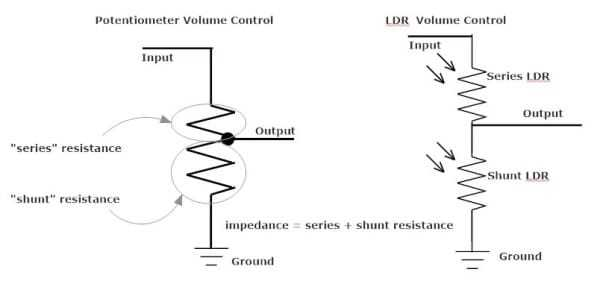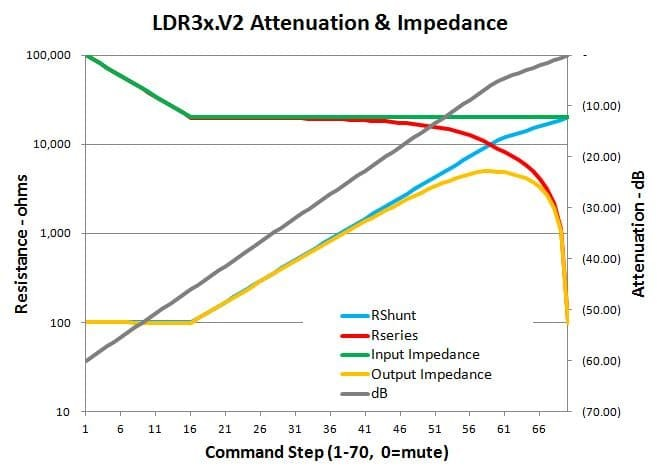# What is Attenuation?

Attenuation is the opposite of amplification.

When you amplify an audio signal you are increasing its average voltage level. When you attenuate an audio signal you reduce its average voltage level. Volume control and attenuation are terms that are often used interchangeably.

Attenuating an audio signal (i.e. reducing its voltage level) is most commonly done using a voltage divider.

The common potentiometer (“pot”) is nothing more than a type of voltage divider.

# How Does Volume Control Work?

Potentiometers used for volume control will have a fixed resistance rating that is typically between 10k and 100k ohms. The audio input signal connects at one end of this fixed resistance and the other end is connected to ground. The output signal from the pot is a third connection to what’s referred to as the “wiper” which slides along the pot’s fixed resistor. The output signal of the pot comes from the wiper.

The resistance above the wiper is the series resistance (Rseries) and the resistance below the wiper is the shunt resistance (Rshunt). The resistance Ratio is defined by the formula: Ratio = Rshunt/(Rshunt + Rseries). This Ratio also happens to be the voltage ratio of Vout divided by Vin such that Vout = Vin x Ratio. Putting all this math together the resulting attenuation expressed in decibels is defined as: dB = 20 x log(Ratio) or if you like dB = 20 x log (Vout/Vin) where Ratio = Vout/Vin = Rshunt/(Rseries + Rshunt).Thus, when Rseries is zero the volume is maximum (no attenuation) and when Rshunt is zero the volume is minimum (maximum attenuation).

Volume control with an LDR is conceptually similar to a potentiometer.

With LDRs, attenuation is achieved by varying the resistance levels of 2 separate series and shunt LDRs to achieve specific resistance ratios that correspond to specific dB attenuation levels. With a pot all this is done mechanically whereas with LDRs it’s done electronically and thus can be manipulated in ways that you can’t with a pot.

# Input Impedance with LDRs

Music is alternating current/voltage (AC), not constant direct current/voltage (DC). With DC electricity we use the term resistance. With AC electricity we use the term impedance. Thus, the term “impedance” is a way of talking about resistance when the voltage is composed of an ever changing voltage over a wide frequency spectrum as is the case with music.

In the section above we defined Rseries and Rshunt. The sum of these 2 values is defined as he nominal impedance of both types of volume control.

If we want to maintain a target 20k ohms input impedance, the combined resistance of the series and shunt LDRs must always equal at least 20k ohms. With LDRs we can’t quite achieve this in practice. With LDRs the Rseries and Rshunt values can never be exactly zero.

For practical reasons such as longevity, we limit the minimum resistance level of our LDRs to 100 ohms and the maximum resistance to 100k ohms. In order to also achieve a full attenuation range of 60 dB within this limited range we have to let the impedance level rise when volume is between 0-22%. At higher volumes the impedance is able to remain constant.

This is illustrated in the graph below of a nominal 20k LDR attenuator. The input impedance (green line) starts at 100k at zero volume, drops down to 20k by step 16 (-46 dB) and than remains constant over the remaining attenuation range. Note that the impedance is the sum of Rseries (red line) and Rshunt (blue line) the ratios of which define the attenuation level in dB (black line) at each step.## Output Impedance

The output impedance of potentiometers and LDR attenuators is neither fixed nor is it independent from the input impedance. As is clear in the above graph, the output impedance (yellow line) varies with each step in the attenuation.

The output impedance is actually the parallel resistance of the series and shunt LDRs. The output impedance starts at ~100 ohm and remains relatively constant until step 16 and then begins to increase along with the shunt resistance. Output impedance peaks at 25% of the nominal input impedance corresponding to step 59 which is at exactly -6 dB of attenuation.

Used as passive attenuators there’s no avoiding the varying output impedance shown. As a practical matter this rarely poses a problem in real world audio applications.

Yes No Suggest edit
Suggest Edit